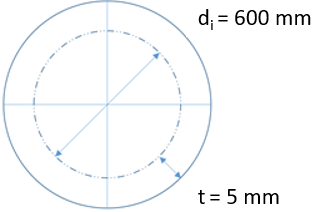## Thin Wall Cylinder

Consider an air cylinder’s pressure gauge reads 2,000 kPa. If the cylinder is made of a 5 mm steel rolled plate, with an internal diameter of 600 mm, what is the tangential stress inside the tank?Hint
The cylinder can be considered thin-walled if:
$$t< \frac{d_o}{20}$$$where $$t$$ is the wall thickness, and $$d_o$$ is the outer diameter. Hint 2 $$\sigma_t=\frac{P_ir}{t}$$$
where $$\sigma_t$$ is the tangential (hoop) stress, $$P_i$$ is the internal pressure, $$r$$ is the mean radius, and $$t$$ is the wall thickness.
The cylinder can be considered thin-walled if $$t< \frac{d_o}{20}$$ where $$t$$ is the wall thickness, and $$d_o$$ is the outer diameter.
$$t< \frac{(600mm+(2)5mm)}{20}=\frac{610mm}{20}=30.5mm$$$Since $$5mm< 30.5mm$$ , the cylinder is thin-walled. Thus, the hoop stress formula is: $$\sigma_t=\frac{P_ir}{t}$$$
where $$\sigma_t$$ is the tangential (hoop) stress, $$P_i$$ is the internal pressure, $$r$$ is the mean radius, and $$t$$ is the wall thickness.
$$r=\frac{r_{inner}+r_{outer}}{2}=\frac{(600mm/2)+[(600mm/2)+5mm]}{2}$$$$$=\frac{300mm+305mm}{2}=302.5mm$$$
Thus, the tangential stress is:
$$\sigma_t=\frac{(2MPa)(302.5mm)}{5mm}=121\:MPa$$\$
121 MPa